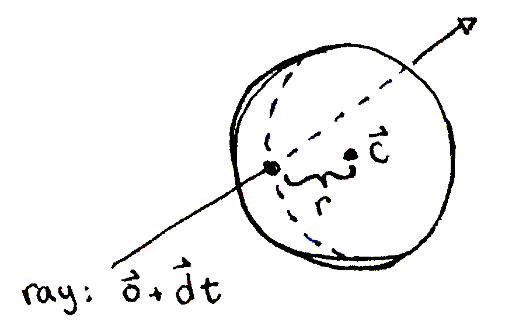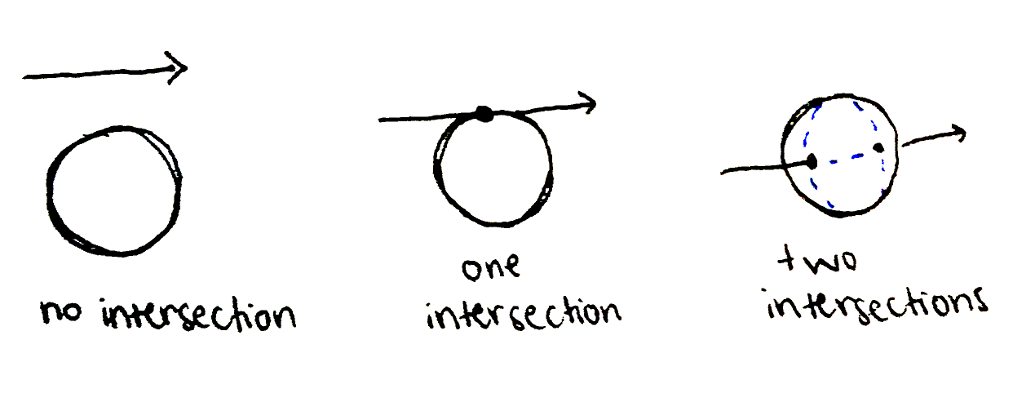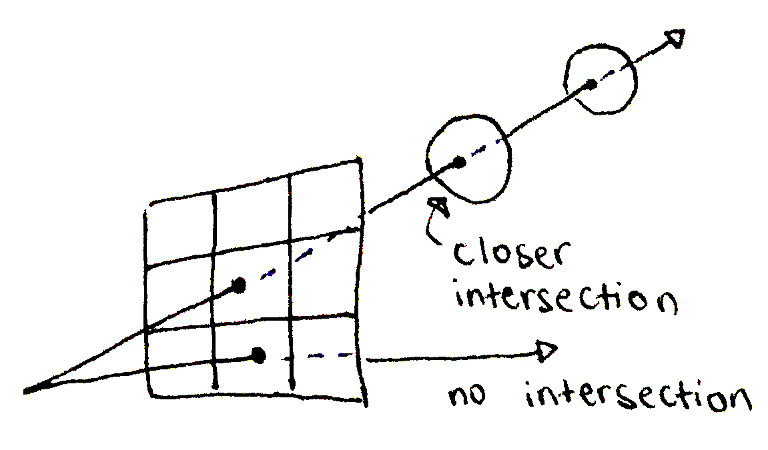# Build your own 3D renderer - Solving the visibility problem with intersection tests

This section deals with vectors norms (magnitudes) and dot products. If you're not familiar with these concepts, or need to brush up, please read the refresher on vectors norms and dot products before reading this section.

How do we know what geometry the camera sees? Answer: one of the rays we cast intersects with the geometry. The closest piece of geometry a particular ray intersects is the piece that will be rendered at the pixel corresponding to that ray.

Triangles are easy to determine intersection for, but spheres are even easier. A sphere is the set of all points for which the distance between that point and the designated center is equal to the radius. Meanwhile, for a ray originating at \vec bbo and going in direction \vec bbd, the ray passes through all the points corresponding to \vec bbo + \vec bbdt, where t >= 0.A ray passes through a point on a sphere, which is a distance r away from the center.

You don't have to understand all of the math below. The project accompanying this section will provide the right formulas to implement in the code. The goal of the derivation below is to show that this process is not magic, and with enough time, you could come up with this yourself.

So, for a point on the ray, the point intersects with the sphere if the distance between the point on the ray and the center vec bbc is equal to the radius r. From this, we solve for t:

\begin{align} dist( \vec{\mathbf{o}} + \vec{\mathbf{d}}t, \vec{\mathbf{c}} ) & = r \\ \Vert \vec{\mathbf{o}} + \vec{\mathbf{d}}t - \vec{\mathbf{c}} \Vert & = r \\ \langle \vec{\mathbf{o}} + \vec{\mathbf{d}}t - \vec{\mathbf{c}}, \vec{\mathbf{o}} + \vec{\mathbf{d}}t - \vec{\mathbf{c}} \rangle & = r^2 \\ \end{align}

As a simplification, we let \vecbbc' = \vecbbo - \vecbbc. Then:

\begin{align} \langle \vec{\mathbf{c}'} + \vec{\mathbf{d}}t, \vec{\mathbf{c}'} + \vec{\mathbf{d}}t \rangle & = r^2 \\ \langle \vec{\mathbf{c}'} + \vec{\mathbf{d}}t, \vec{\mathbf{c}'} \rangle + \langle \vec{\mathbf{c}'} + \vec{\mathbf{d}}t, \vec{\mathbf{d}}t \rangle & = r^2 \\ \langle \vec{\mathbf{c}'}, \vec{\mathbf{c}'} \rangle + \langle \vec{\mathbf{d}}t, \vec{\mathbf{c}'} \rangle + \langle \vec{\mathbf{c}'}, \vec{\mathbf{d}}t \rangle + \langle \vec{\mathbf{d}}t, \vec{\mathbf{d}}t \rangle & = r^2 \\ \Vert \vec{\mathbf{c}'} \Vert ^ 2 + t^2 \Vert \vec{\mathbf{d}} \Vert ^ 2 + 2t \langle \vec{\mathbf{c}'}, \vec{\mathbf{d}} \rangle & = r^2 \\ \left( \Vert \vec{\mathbf{d}} \Vert ^ 2 \right) t^2 + \left( 2 \langle \vec{\mathbf{c}'}, \vec{\mathbf{d}} \rangle \right) t + \left( \Vert \vec{\mathbf{c}'} \Vert ^ 2 - r^2 \right) & = 0 \end{align}

Apply the quadratic equation to find t. The math is explained in the accompanying project. The possibilities are:

• No real t satisfies the equation ⇒ ray does not intersect sphere.
• One value of t satisfies the equation ⇒ ray grazes sphere, considered a hit.
• Two values of t satisfies the equation ⇒ ray passes through sphere. Pick the smaller value to find the location closer to the camera.A ray might not interspect the sphere, or it might intersect it at one or two points.

Remember that when t = 1, \vec bbo + \vec bbdt = \vec bbo + \vec bbd = \vec bbo + (\vec bbp - \vec bbo) = \vec bbp, which is on the image plane. So, in all of the above scenarios, we will only consider any t >= 1, where the intersection is past the image plane.

For a single ray, we'll go through each piece of geometry in the scene, testing each object for an intersection. Out of all the intersections for a single ray, we pick the one closest to the camera (but still past the image plane), and the object corresponding to that hit determines the color of the pixel associated with the ray. If a ray does not intersect any geometry, the corresponding pixel is painted a default background color, say black.A ray passes through multiple objects, but the farther intersections are blocked by the closer ones visually.